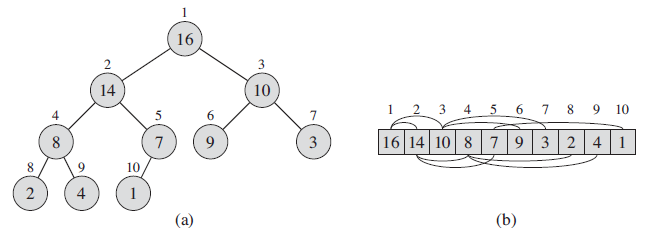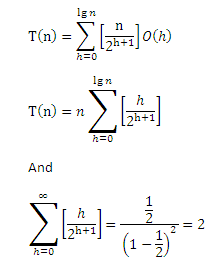# Implementation of Heap Sort with C# and analysis of running time on best, worst and average case

 Category: Data Structure And Algorithms Tags:heap sort code files

## Introduction

As we seen analysis of merge sort in previous article which complexity was O(n log n). In this article we are going to analyze one more algorithm called heap sort which runs with same execution time O(n log n) but this algorithm is easy to implement and we introduce one data structure called heap data structure similar to binary tree.

Heaps:- heap data structure is an array object that can be viewed as binary tree as shown in figure below, each node is an element of array. A heap object has two attributes one is Length and other one is Size where the Length is the number of elements in array and Size is how many elements of heap are stored actually in array.Image Reference: Introduction To Algorithms Book(CLRS)

In above diagram we have a max-heap, a max-heap in which the children's node value is less than root's value and the top element is the largest one, In figure(b) we shown the array representation of heap. The min-heap is right opposite to max-heap where children will always be greater than root.

Index Calculation:- here we can simply calculate the root's index if child index is given or we can calculate children index if root's index is given, like:

1. If root's index is 2 so children's index will be 4 (2*2 = 4) which is left child and (2*2 + 1 = 5) which is right child as shown in figure(b)
2. And for parent's index we can simply divide child's index by 2, like child's index is 4 so it's parent's index will be 2 (4/2 = 2).

## Implementation & Analysis

To implement heap sort we need to go through 3 steps where we will create one method in each step and each step performs a specific operation on an array. Here we will make use of Max-Heap and the class structure will be like this:

`public class Heap{    public int[] A;    public int heapSize; // mostly will give the index of last element in heap    //constructor    public Heap(int[] array)    {        A = array;        heapSize = array.Length - 1;    }    //returns left child's index    public int ChildL(int parent)    {        return parent * 2 + 1;    }    //returns right child's index    public int ChildR(int parent)    {        return (parent * 2 + 2);    }    //swaps two index values in an array    public void Swap(int i, int j)    {        if (i <= heapSize && j <= heapSize && i != j)        {            int temp = A[i];            A[i] = A[j];            A[j] = temp;        }    }    public void MaxHeapify(int i){...}    public void BuildHeap(){...}    public void HeapSort(){...}}`

Step 1(Maintaining Max-Heap): We have to create a method called MaxHeapify which will be used to maintain the maximum heap, we can give any index i and from index i execution will go forward to its children and balance the heap until we reach at end of the array. The implementation of MaxHeapify given below:

```/// <summary>
/// balance the heap, expect parameter i which is index of any element
/// from you want to balance heap till end
/// </summary>
/// <param name="i"></param>
public void MaxHeapify(int i)
{
int l = ChildL(i);
int r = ChildR(i);
int largest = i;
if (l <= heapSize && A[l] > A[i])
largest = l;
if (r <= heapSize && A[r] > A[largest])
largest = r;
if (largest <= heapSize && largest != i)
{
Swap(largest, i);
MaxHeapify(largest);
}
}
```

The above method finds if there any bigger value is available in children nodes then it will replace the root with child and that's how it maintains maximum heap.

The above figure(a) we can see the heap is having similar structure of binary tree and method MaxHeapify runs through root to children and from children to their children and so on, so the complexity of this method will be O(lg n) or O(h) where h is height of node in tree.

Step 2(Build Max-Heap): Let's suppose we have given an array with n elements which are in random order. BuildHeap do arrange the elements in heap structure and maintains max-heap property.

```public void BuildHeap()
{
for (int i = (A.Length / 2); i >= 0; i--)
{
MaxHeapify(i);
}
}
```

In above method we can see we are running the loop from mid of the array to start index. Which will balance this array in max heap. only from mid is required because A[n/2+1 .... n] all are leafs in the heap, we only need parent nodes to balance the heap.

For complexity calculation we can say MaxHeapify has complexity O(lg n) and which loops out n times here so the complexity would be O(n lg n) and that is not asymptotically tight, to calculate accurate complexity:

Suppose we start loop from node zero(consider figure a) where height will be lg(n) and only one node is there at this height, if we go one step down at height lg(n)-1 we see the nodes at this level are two, so we can say we have approximate nodes at height h are [n/2h+1]. And we already proven that complexity of MaxHeapify is O(h) so complexity of BuildHeap can be given as:So we can say T(n) = n . 2 = O(n) which is asymptotically tight.

Step 3(Heap Sort): Finally, we are ready to sort the array, only one more method is required which will re-arrange the elements. Because our top element is the biggest in max-heap, we will start replacing this element with bottom elements and will call MaxHeapify in every iteration with reducing the size of heap to sort in increasing order:

```public int[] HeapSort(){    BuildHeap();    for (int i = heapSize; i > 0; i--)    {        Swap(i, 0);        heapSize--;        MaxHeapify(0);    }    return A;}
```

Here we are just placing big elements to end and balancing the heap in every iteration the loop which is running from Size(attribute of heap described in introduction) to 1, not up to 0. Let's run the above code:

`Heap heap = new Heap(new int[] { 2, 1, 7, 4, 9, 3, 6 });var sortedArr = heap.HeapSort();foreach (int ele in sortedArr)    Console.WriteLine(ele);Console.ReadLine();`

Output:

1

2

3

4

6

7

9

BuildHeap is already calculated which is O(n) and the loop is just replacing and running from 1 to Size (total n-1 times which will be O(n) ) with MaxHeapify method inside which complexity is O(lg n) so:

T(n) = O(n) + O(lg n) O(n)

=> T(n) = O(n lg n)

Hear we reached on conclusion that Heap Sort runs on complexity O(n lg n).

 Like2 PeopleNikhil Joshi Ceo & Founder at Dotnetlovers Atricles: 158 Questions: 16 Given Best Solutions: 16 *Keep writing blogs on algorithms, they are really helpful.
Like1 Person on 9 February 2016

Login via:x